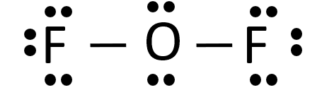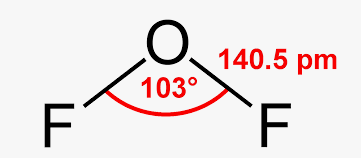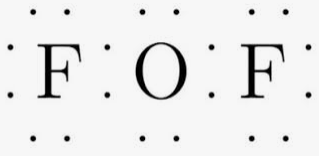of2 lewis structure homework Help at TutorEye

# Best Homework Help For of2 lewis structure

## Top Questions

erature and pressure? Record your answer in scientific notation using 3 significant figures.
View More

### What is the Lewis structure of OF2?

Each fluorine atom has 7 valence electrons and oxygen atom has 6 valence electrons, total valence electrons of OF2 molecule is 20.
Oxygen atom share two electrons each with both fluorine atoms to form a single covalent bond each with two F atoms, each O-F bond is represented by a solid line between O and F. The three pairs of dots on both F and two pairs of dots on O represent lone pairs.
Both fluorine and oxygen are surrounded by eight electrons thus complete octet.### What is the molecular shape of OF2?

Due to the presence of two lone pairs on the oxygen which is the central atom causing repulsion with bond pairs of electrons, as a result, the fluorine atoms pushes down to minimize the repulsions making the molecule bent. The repulsions between lone pairs of F also widens the angle.### How many nonbonding pairs are in OF2?

Each fluorine atom has 7 valence electrons and oxygen atom has 6 valence electrons, total valence electrons of OF2 molecule is 20. Oxygen uses two valence electrons to form two single bonds with two F atoms and is left with 4 valence electrons or two lone pairs. Each F shares its one valence electron with oxygen to form a single bond and is left with 6 valence electrons or three lone pairs.
Total nonbonding electrons in OF2 are 16, and thus total non-bonding pair are 8.

### What is the lewis dot structure for OF2?

Oxygen atom share two electrons each with both fluorine atoms to form a single covalent bond each with two F atoms, each O-F bond is represented by two dots between O and F. The three pairs of dots on both F and two pairs of dots on O represent lone pairs.
Both fluorine and oxygen are surrounded by eight electrons thus complete octet.